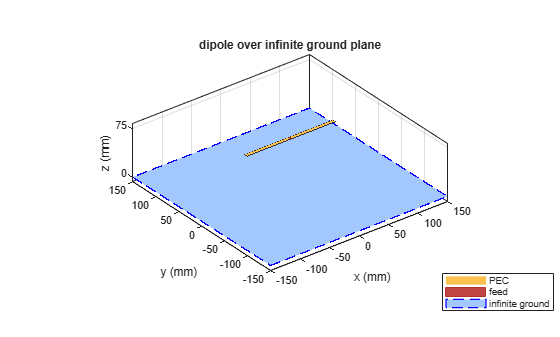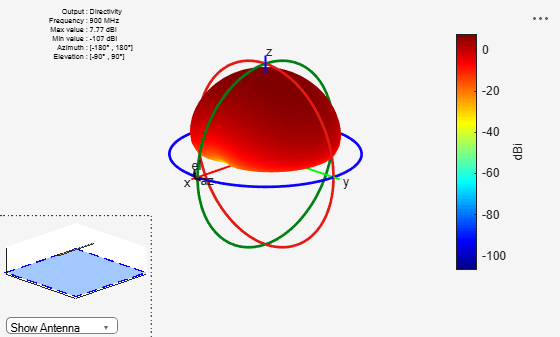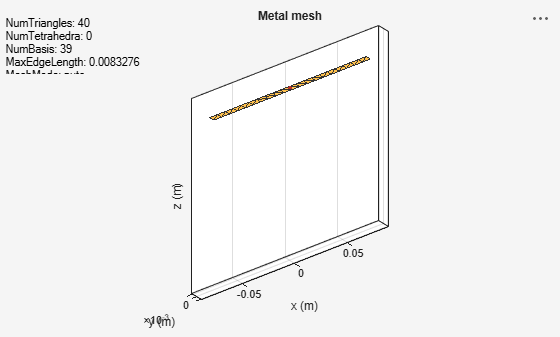Documentation

## Model Infinite Ground Plane for Balanced Antennas

This example shows how to model an infinite ground plane and calculate fundamental antenna parameters for balanced antennas.

### Create Antenna On Infinite Ground Plane

Create a reflector antenna on a ground plane of infinite length.

`r = reflector ('GroundPlaneLength', inf)`
```r = reflector with properties: Exciter: [1x1 dipole] Substrate: [1x1 dielectric] GroundPlaneLength: Inf GroundPlaneWidth: 0.2000 Spacing: 0.0750 EnableProbeFeed: 0 Tilt: 0 TiltAxis: [1 0 0] Load: [1x1 lumpedElement] ```

### View Antenna Geometry

View the physical construction of infinite ground plane reflector antenna.

`figure;show(r);`### Calculate Impedance of Antenna

Calculate the impedance of reflector antenna over a frequency range of 800MHz to 1GHz.

`figure;impedance(r,800e6:5e6:1e9);`### Plot Radiation Pattern of Antenna

Plot the radiation pattern of reflector antenna at a frequency of 900MHz.

`figure;pattern(r,900e6);`### Visualize Antenna Mesh

Mesh and view the infinite ground plane reflector antenna.

`figure; mesh(r);`